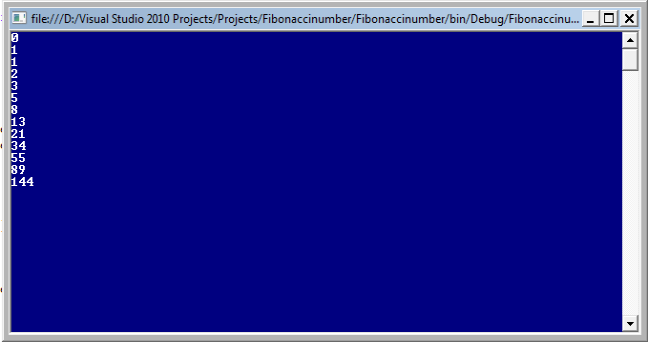Featured

# Print Fibonacci Numbers Below 200 Using C#

I will explain in this article how to print the numbers in  using C#, this type of question might be asked by an interviewer in a .Net position related interview.

My intent for this article is to explain how to answer a question that is often asked in an interview, which is: Write a program to print the Fibonacci series of a given number. A candidate new to the interview can become totally confused because the first problem is the candidate does not even know what a Fibonacci number is, so how will he or she know how to write the program?

So considering the above requirement I have decided to write this article with the basics and specially focusing on the beginner, student and whoever might face this type of question in an interview.

What are Fibonacci numbers?

The number in a series which is the sum of his previous last two numbers from the left are called Fibonacci numbers.

Example
Suppose the following series of Fibonacci numbers:

0 0 1 1 2 3 5 8 13 21

See the above given Fibonacci numbers and closely observe them from the left how the numbers are represented, in other words each number is a sum of the previous last two numbers from the left. These types of numbers are called Fibonacci numbers.

Now that we completely understand what Fibonacci numbers are, next I will explain how to do it step-by-step, as in the following:
1. Open Visual Studio from Start - - All programs -- Microsoft Visual Studio.
2. Then go to to "File" -> "New" -> "Project..." then select Visual C# -> Windows -> Console application.
3. After that specify the name i.e Fibonacci number or any name as you wish and the location of the project and click on the OK button. The new project is created.
And use following code in the program.cs class file:

using System;

namespace Fibonaccinumber
{
class Program
{
static void Main(string[] args)
{

int a=0;     // assigning initial value for first variable
int b=1;     // assigning initial value for second  variable
int c=1;     // assigning initial value for third variable

Console.WriteLine(a);
Console.WriteLine(b);
while (true)
{

c = a + b;
if (c >= 200)
{
break;
}
Console.WriteLine(c);
a = b;
b = c;

}
Console.Read();  // to keep window screen after running

}
}
}

The output of the above program will be as:From the above output it is clear that,"the numbers in a series which is the sum of  the previous two numbers from the left are called Fibonacci numbers".

I hope it is useful for all beginners and other readers.